• No products in the cart.

# K-Means Clustering in Python using sklearn

## Introduction

Do you know how huge marketing firms target products to specific customers who might be interested in them? How do they make specific marketing campaigns for a particular group of customers? Well, it’s no rocket science and you can also achieve this easily using customer segments.

Customer segments are nothing but a bunch of customers grouped who have similar interests and needs. They help separate a certain group of people from the rest which essentially lets companies focus on the particular people they want to, in order to generate revenues.

Clustering is the principle used behind all this. It’s an unsupervised learning technique used when labeled data is not available. K-Means clustering is a special type of clustering algorithm that can take in data without any labels and output the labels of the data depending upon its features.

In this article, we’ll be learning about how you can easily implement K-Means Clustering using sklearn library. So, let’s dig in without any further ado.

## What is K-Means Clustering?

K-Means Clustering is amongst the simplest clustering algorithms out there. The algorithm takes in the value of K and makes K number of clusters of the data provided. The algorithm is unsupervised, so you don’t need to have labeled data to use this algorithm.

The value of K in this algorithm plays a pivotal role. As you will see further in the article, it can make or break the performance of the algorithm. However, there’s no definite rule on what value of K could provide you the optimum results. The only solution is to change the number of clusters (the value of K) and compare the results to find the optimal number of clusters for the dataset. However, some custom methods can be used to estimate the optimal numbers, but it’s not guaranteed that they’ll always work.

Moreover, K-Means clustering is a very fast algorithm with a very less computational cost, making it feasible for a wide range of applications such as language clustering, article clustering, and even anomaly detection.

## Problem Definition

We will be using the iris dataset to implement K-Means Clustering in this article. The dataset contains the features of different iris plants and we will be clustering the data points into the respective type of plants using the features provided in the data. Note that we do not have any idea of how many clusters we need to make, and we cannot refer to the target class of the dataset since this is an unsupervised learning algorithm.

## Implementing the Algorithm

The iris dataset I mentioned before comes with 3 classes having 50 instances each. It has data about 3 different species of iris plants. Even though the dataset here is labeled, we don’t need the labels of the dataset while implementing the algorithm, but they are beneficial as we can compare our clusters in the end with the total classes in the dataset. If the clusters we end up making equal the number of classes in the original dataset, we can be sure that we have clustered the data perfectly.

Before we start, let me give a brief overview of how the algorithm works. Below is the high-level view of the algorithm showing the major steps involved.

-Assume we have a list of data as points that need to be clustered i.e. a1, a2, a3

Step 1: Pick a random value of K, as the total number of clusters to initialize.

Step 2: Assign each data point ai to it’s nearest cluster by calculating the distance to the centroid

Step 3: Find new clusters by averaging all the new points assigned to a cluster.

Step 4: Keep repeating steps 2 and 3 until clusters don’t change.

Now that we have seen how the algorithm works, let’s jump on to the coding part and see how easy it is to implement the algorithm using sklearn.

First, we need to import all the libraries that we need to read and manipulate the data. Here are all the libraries we need.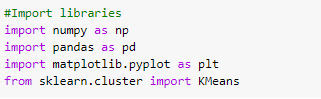?

Now, we will use the read_csv method of the Pandas library to read our iris dataset. Make sure you download the dataset from this link first before importing it to the notebook: https://archive.ics.uci.edu/ml/datasets/Iris

Once we have imported the dataset to our notebook, we can then print out its head using the df.head() method of Pandas to see how the first few columns of the dataset look like.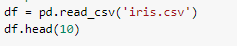?

Here are the results of the query.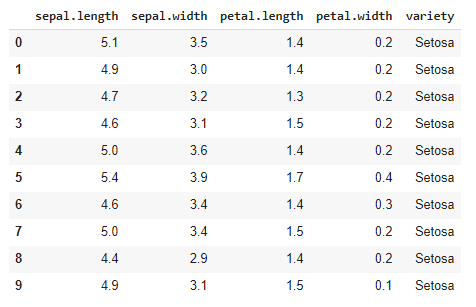?

As you can see, there are 4 different features i.e. ‘Sepal Length’, ‘Sepal Width’, ‘Petal Length’, and ‘Petal Width’ that help us identify the variety or ‘species’ of the plant. In the data head shown above, all the plans belong to the same species ‘Setosa’ of the iris plant.

Since we need to train our algorithm on the four features that we saw above, we need to separate those features in an array to later pass them to our model. Let’s use a variable called ‘features’ and put the features in it.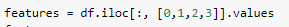?

Here’s how the array looks like.?

Now, we need to make an instance of the K-Means algorithm from the KMeans class and randomly pass a value of K to the function. We will start with the value of K=5 and pass the training data that we made in the previous step so the model can train on it.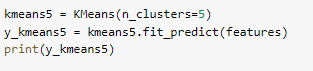?

Upon running this print query, we can see how the algorithm clustered the data points based on their features.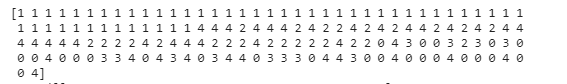?

The numbers shown above represent the clusters to which the data points belong, as calculated by the algorithm. We can also see the centers of final clusters made by the algorithm. The function for viewing the centers is clusters_centers_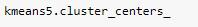?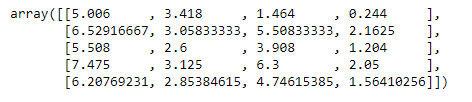?

As you can see, there are 5 centroids, each of which corresponds to their respective clusters. Note that there are 5 centroids because we initially passed the value of K as 5.

However, we don’t know if the clusters are made optimally and if there is room for more improvement. So, how do we know if we needed to make more or fewer clusters to perfectly cluster the data into appropriate classes? Well, turns out that we have several methods that can help us estimate the optimal number of clusters according to a dataset. One such method is called the elbow method, which we will be using here.

The Elbow method essentially plots a graph of the number of clusters against the total error in the output. Once the graph is made, we simply have to spot the ‘elbow’ shape on the graph and the place where this shape appears is usually the optimal number of clusters to make. Let’s see how the elbow method can be coded.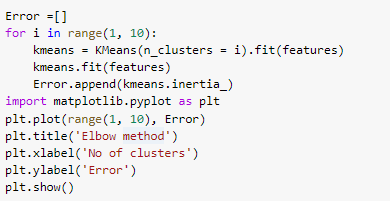?

Let’s run this code to see the output elbow graph.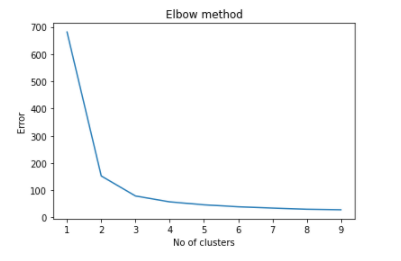?

If you noticed, the ‘elbow’ shape is clearly visible between the value of 2 and 4 (the turning point of the graph). So, we can be sure of the fact that the optimal number of clusters for this dataset is at K=3 since it’s the only number between 2 and 4. So, let’s repeat the process again and implement the algorithm using K=3.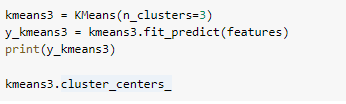?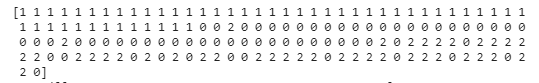?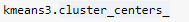?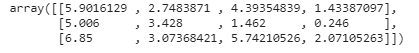?

As we have finally made the optimum number of clusters for this dataset, it’s time for some data visualization now. It will help us see how well the three clusters are actually divided and whether they are overlapping or not. We will represent each cluster with a different color so they don’t mix up into each other.

To plot this visualization showing the clusters, we will use scatter plot using the matplotlib library.?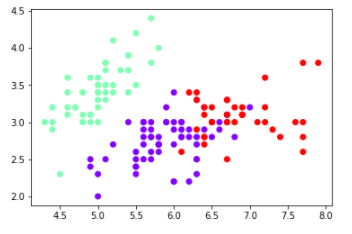?

Using this scatter plot, we can see how well the clusters are divided and since the original target classes were also 3, we have successfully found the optimal number of clusters for this problem without using the labels of data.

## Summary

K-Means Clustering is a very intuitive and easy to implement an unsupervised learning algorithm. Throughout the article, we saw how the algorithm can be implemented using ‘sklearn.cluster’ library. Even though you can also code the algorithm from scratch using the pseudocode I showed above, using sklearn is a much easier and quicker way. Moreover, we saw how the elbow method helps us find the optimal number of clusters to make for a specific dataset. In the end, we visualized the clusters as well to see how well is the dataset separated into clusters.

Lastly, if you want to access the complete code used in this tutorial, you can get it from my GitHub.

August 9, 2021